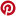# Dilation Transformation

Related Topics:
More Geometry Lessons
Transformation Games

In these lessons, we will learn
• what is dilation or enlargement and reduction?
• dilation with scale factor greater than 1.
• dilation with scale factor between 0 and 1.
• dilation with a negative scale factor.
• dilation on the coordinate plane.

### What is Dilation or Enlargement?

A dilation is a transformation that produces an image that is the same shape as the original, but is a different size. (The image is similar to the original object). Dilation is a transformation in which each point of an object is moved along a straight line. The straight line is drawn from a fixed point called the center of dilation. The distance the points move depends on the scale factor. The center of dilation is the only invariant point.

Scale factor =If the scale factor is greater than 1, the image is an enlargement.
If the scale factor is between 0 and 1, the image is a reduction.

Example:

The figure shows two similar triangles PQR and P’Q’R’. Triangle P’Q’R’ is a dilation of triangle PQR. We say that triangle PQR is transformed onto triangle P’Q’R’ by a dilation with center at O and scale factorThe following diagrams show the triangle ABC dilated with different scale factors. Scroll down the page for more examples and explanations of dilations.### Dilation with scale factor > 1

We will first look at enlargements which are dilations with scale factors greater than 1

Example :

Enlarge triangle PQR with O as the center of dilation and a scale factor of 2.Solution:

Step 1: Measure OP.
Step 2: Extend the line OP to the point P’ such that OP’ = 2OP.
Step 3: Repeat the steps for all the vertices: point Q to get Q' and point R to get R'.
Step 4: Join the points P’Q’R’ to form the image.Example:Enlarge triangle ABC with C as the center of dilation and a scale factor of 3.

Solution:

Step 1: Measure CA.
Step 2: Extend the line CA to the point A’ such that CA’ = 3CA.
Step 3: Repeat the steps for point B to get B'.Note that in this example, all the points in the triangle have been transformed except point C, which is the only invariant point.

Example:

Draw an image of the figure PQRS. O is the center of dilation and the scale factor is 1.5.Solution:

Step 1: Join OP.
Step 2: Extend the line OP to OP’, such that OP’ = 1.5 × OP
Step 3: Repeat for all the other vertices Q, R and S.
Step 4: Join P’, Q’, R’ and S’ to form the image.An introduction to the concepts of dilation transformations
What happens when the scale factor or the center of the dilation is changed?

### Dilation with scale factor between 0 and 1

If the scale factor of a dilation is between 0 and 1, the image will be smaller than the object. It is then called a reduction.

Example :

Enlarge triangle PQR with O as the center of enlargement and scale factor.Solution:

Step 1 : Join O to P.
Step 2 : Mark off the point P ’ on OP such that OP' =OP.
Step 3 : Repeat the steps for all the vertices: point Q to get Q' and point R to get R'
Step 4 : Join the points P'Q'R' to form the image.Dilation of a Geometric Figure
How to create a dilation of a geometric figure using a center of dilation and a scale factor of half?

### Dilation with a Negative Scale Factor

If the scale factor of a dilation is negative then the image will be on the opposite side of the center of dilation compared with the object.

How to create a dilation of a geometric figure using a center of dilation and a negative scale factor?

### Dilation on the Coordinate Plane

We will now look at how to create a dilation on a coordinate plane.

Dilations
A dilation is a non-rigid transformation, which means that the original and the image are not congruent. They are, however, similar figures.
To perform dilations, a scale factor and a center of dilation are needed. If the scale factor is larger than 1, the image is larger than the original; if the scale factor is less than 1, the image is smaller than the original. How to get the scale factor given the points of the shape and its image?

Rotate to landscape screen format on a mobile phone or small tablet to use the Mathway widget, a free math problem solver that answers your questions with step-by-step explanations.

You can use the free Mathway calculator and problem solver below to practice Algebra or other math topics. Try the given examples, or type in your own problem and check your answer with the step-by-step explanations.